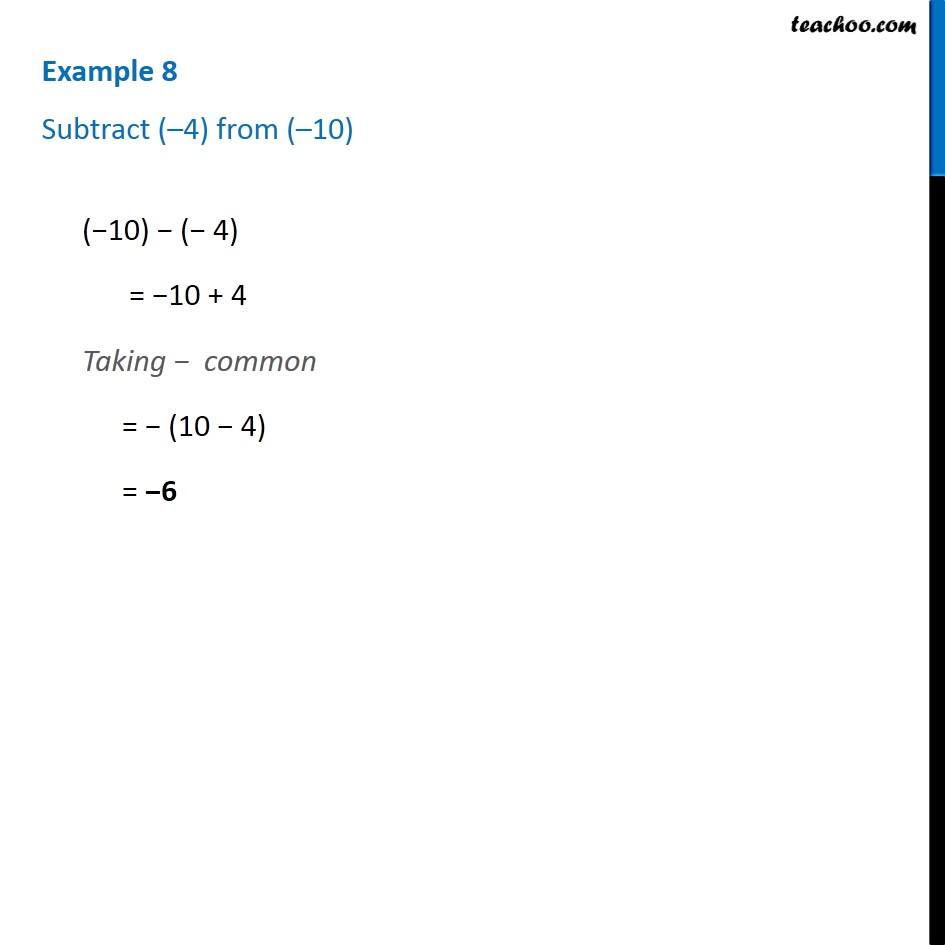1. Chapter 6 Class 6 Integers
2. Concept wise
3. Subtraction of integers

Transcript

Example 8 - Chapter 6 Class 6 Integers - NCERT Subtract (–4) from (–10) (−10) − (− 4) = −10 + 4 Taking − common = − (10 − 4) = −6

Subtraction of integers

Chapter 6 Class 6 Integers
Concept wise